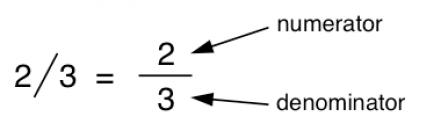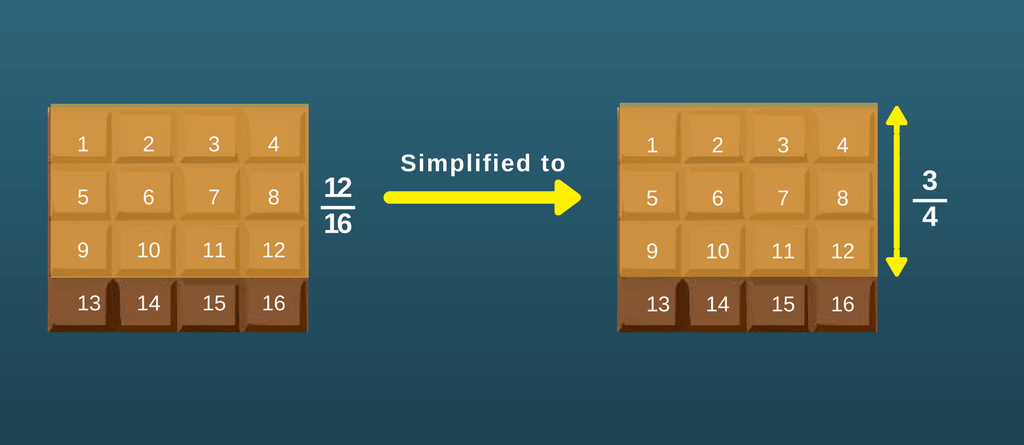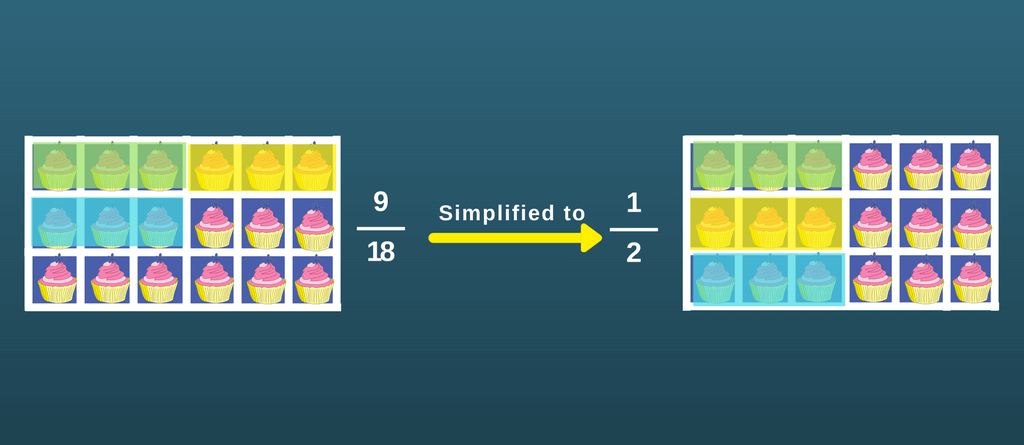Simplifying fractions has been one of the major concern for most children. But, worry not! There is an easy explanation to it and we have it right here. So, let’s go slow.

## What is a Fraction

A Fraction is a part of a whole or a unit, for eg. A piece of a whole cake (1â„2 of 1 cake).

A simple fraction consists of two parts, a Numerator and a Denominator.## How to simplify fractions

Here we explain the quickest way to determine how to simplify fractions. This process will take less than a minute to derive to the simplest form. Understand the quick trick.

### Step 1 -Â Find the commonÂ factor

When you see a fraction, you see a numerator and a denominator. To find the common factor, seeÂ whichever number among these is least:

The numerator, the denominator or the difference between numerator and denominator.

### Step 2 -Â Divide by the factor found

Now simply divide the fraction with the least number found to getÂ your answer.

Let’s work on few examples to make it more clear:

Example 1: Simplifying fractions -Â 12/16:

 12 16

### Step 1 -Â Find the commonÂ factor

The numerator – 12

The denominator – 16

Difference between numerator and denominator: 16 – 12 = 4

Yes! 4 is the least among them.

### Step 2 -Â Divide by the factor found

Now, let’s divide the fraction by 4.

 12 = 3 16 4

Since, 12 Ã· 4 = 3 and 16 Ã· 4 = 4.

Now the fraction is 3/4. Since the least factor is 1 (difference between numerator and denominator), it cannot be further simplified. Hence, 3/4 is in it’s lowest form of fraction.Isn’t simplifying fractions easy? Save lot of time with this super fast trick. Let’s explore more:

Example 2: Simplifying fractions – 50/8:

 50 8

### Step 1 -Â Find the commonÂ factor

The numerator – 50

The denominator – 8

Difference between numerator and denominator: 50 – 8 = 42

Yes! 8 is the least among them.

### Step 2 -Â Divide by the factor found

Now,Â we know that 8 is the least number. But here, 50 is not a factor of 8. So, we see the factors of 8 – 1, 2, 4 and 8.

Now, 2 is a common factor of both 50 and 8. Seems perfect. Let’s divide the fraction by 2.

 50 = 25 8 4

Since, 50 Ã· 2 = 25 and 8 Ã· 2 = 4.

So if the least among the three does not work, donâ€™t forget to check outÂ its factors.

Now the fraction is 25/4. Since the least factor is 4 (denominator), it cannot be further simplified. 25 is not divisible by 4 or a factor of 4. Â Hence, 25/4 is in it’s lowest form of fraction.

Example 3: Simplifying fractionsÂ – 9/18

 9 18

### Step 1 -Â Find the commonÂ factor

The numerator – 9

The denominator – 18

Difference between numerator and denominator: 18 – 9 = 9

9 is the least among them.

### Step 2 -Â Divide by the factor found

Now, let’s divide the fraction by 9.

 9 = 1 18 2

Since, 18 Ã· 9 = 2.

Now the fraction is 1/2 and it cannot be further simplified.Example 3: Simplifying fractionsÂ – 60/32

 60 32

### Step 1 -Â Find the commonÂ factor

The numerator – 60

The denominator – 32

Difference between numerator and denominator: 60 – 32 = 28

So, 28 is the least among them.

### Step 2 -Â Divide by the factor found

Now,Â we know that 28 is the least number. But here, 60 and 32 are not the factors of 28. So, we see the factors of 28 – 1, 2, 4, 7, 14 and 28.

Now, 2 and 4, both are the common factors of both 60 and 32. Let’s divide the fraction by 4.

 60 = 15 32 8

Since, 60 Ã· 4 = 15 and 32 Ã· 4 = 8.

Remember -Â If the least among the three does not work, donâ€™t forget to check outÂ its factors.

Now the fraction is 15/8. Since the least factor is 7 (difference between numerator and denominator), it cannot be further simplified. Neither 15 nor 8 is divisible by 7 or a factor of 7. Â Hence, 15/8 is in it’s lowest form of fraction.

Example 4: SimplifyingÂ fractions – 9/15:

 9 15

### Step 1 -Â Find the commonÂ factor

The numerator – 9

The denominator – 15

Difference between numerator and denominator: 15 – 9 = 6

6 is the least among them.

### Step 2 -Â Divide by the factor found

Since 15 and 9 are not divisible by 6, factors of 6 (1, 2, 3, 6) can be tried. Among all the factors, 3 seem to be the common one.

 9 = 3 15 5

Since, 15 Ã· 3 = 5 and 9 Ã· 3 = 3.

Now the fraction is 3/5. Since the least factor is 2 (difference between numerator and denominator), it cannot be further simplified. Hence, 3/5 is in it’s lowest form of fraction.

Example 5: Simplifying fraction 28/38:

 28 38

### Step 1 -Â Find the commonÂ factor

The numerator – 28

The denominator – 38

Difference between numerator and denominator: 10

### Step 2 -Â Divide by the factor found

Now, 28 and 38 are not divisible by 10. But among factors of 10 (1, 2, 5, 10), 2 seems perfect. So let’s divide by 2.

 28 = 14 38 16

Since, 28 Ã· 2 = 14 and 38 Ã· 2 = 19.

Now the fraction is 14/19. Can it be further simplified? Let’s see.
Numerator – 14
Denominator – 19
Difference – 5
5 being the lowest and is not factor of both, this fraction cannot be further simplified. So,

 14 19

## Simplifying Fractions – More Resources

This was the step by step solution for simplifying fractions, Â hope it made yourÂ calculations easy. But why stop here? Get more and more fun practice, not from text books but from math games and FREE math worksheets.

Here’s hoping that now simplifying fractions for you would be easy and not so ‘trick-y’.Â Happy Fractions!